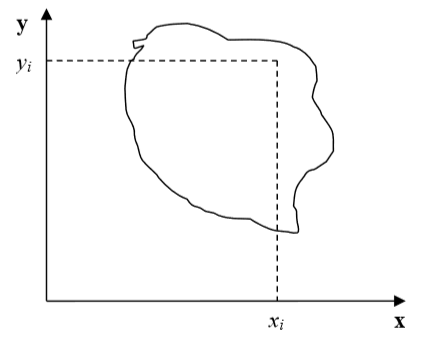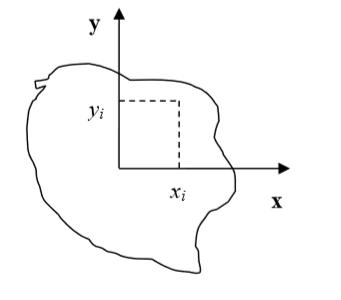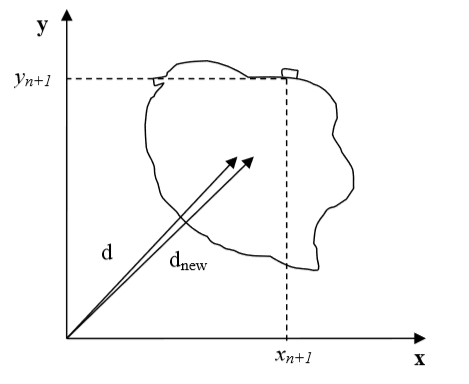# Calculating Inertia tensor In CC3D¶

For each cell the inertia tensor is defined as follows:

\begin{eqnarray} I = \begin{bmatrix} \sum_i y_i^2+z_i^2 & -\sum_i x_i y_i & -\sum_i x_i z_i \\ -\sum_i x_i y_i & \sum_i x_i^2+z_i^2 & -\sum_i y_i z_i \\ -\sum_i x_i z_i & -\sum_i y_i z_i & \sum_i x_i^2+y_i^2 \\ \end{bmatrix} \end{eqnarray}

where index $$i$$ denotes i-th pixel of a given cell and $$x_i$$, $$y_i$$ and $$z_i$$ are coordinates of that pixel in a given coordinate frame.Figure 3: Cell and coordinate system passing through center of mass of a cell. Notice that as cell changes shape the position of center of mass moves.Figure 4: Cell and its coordinate frame in which we calculate inertia tensor

In Figure 4 we show one possible coordinate frame in which one can calculate inertia tensor. If the coordinate frame is fixed calculating components of inertia tensor for cell gaining or losing one pixel is quite easy. We will be adding and subtracting terms like $$y_i^2+z_i^2$$ or $$x_i z_i$$.

However, in CompuCell3D we are mostly interested in knowing tensor of inertia of a cell with respect to xyz coordinate frame with origin at the center of mass (COM) of a given cell as shown in Figure 3. Now, to calculate such tensor we cannot simply add or subtract terms $$y_i^2+z_i^2$$ or $$x_i z_i$$ to account for lost or gained pixel. If a cell gains or loses a pixel its COM coordinates change. If so then all the $$x_i$$,:math:y_i, $$z_i$$ coordinates that appear in the inertia tensor expression will have different values. Thus, for each change in cell shape (gain or loss of pixel) we would have to recalculate inertia tensor from scratch. This would be quite time consuming and would require us to keep track of all the pixels belonging to a given cell. It turns out, however, that there is a better way of keeping track of inertia tensor for cells. We will be using parallel axis theorem to do the calculations. Parallel axis theorem states that if $$I_{COM}$$ is a moment of inertia with respect to axis passing through center of mass then we can calculate moment of inertia with respect to any parallel axis to the one passing through the COM by using the following formula:

\begin{eqnarray} I_{x'x'} = I_{xx} + Md^2 \end{eqnarray}

where $$I_{xx}$$ denotes moment of inertia with respect to x axis passing through center of mass, $$I_{x'x'}$$ is a moment of inertia with respect to some other axis parallel to the x , d is the distance between the axes two and M is mass of the cell.

Let us now draw a picture of a cell gaining one pixel:Figure 5: Cell gaining one pixel.d denotes a distance from origin of a fixed frame of reference to a center of mass of a cell before cell gains new pixel. $$d_{new}$$ denotes same distance but after cell gains new pixel

Now using parallel axis theorem we can write expression for the moment of inertia after cell gains one pixel the following that:

\begin{eqnarray} I_{xx}^{new} = I_{x'x'}^{new} - (V+1)d_{new}^2 \end{eqnarray}

where, as before, $$I_{xx}^{new}$$ denotes moment of inertia of a cell with new pixel with respect to x axis passing through center of mass, $$I_{x'x'}^{new}$$ is a moment of inertia with respect to axis parallel to the x axis passing through center of mass, $$d_{new}$$ is the distance between the axes and $$V+1$$ is volume of the cell after it gained one pixel. Now let us rewrite above equation by adding ad subtracting $$Vd^2$$ term:

\begin{eqnarray} I_{xx}^{new} = I_{x'x'}^{old} + y_{n+1}^2 + z_{n+1}^2 - Vd^2 + Vd^2 (V+1)d_{new}^2 \\ = I_{x'x'}^{old} - Vd^2 + y_{n+1}^2 + z_{n+1}^2 + Vd^2 (V+1)d_{new}^2 \\ I_{xx}^{old} - Vd^2 + y_{n+1}^2 + z_{n+1}^2 + Vd^2 (V+1)d_{new}^2 \end{eqnarray}

Therefore we have found an expression for moment of inertia passing through the center of mass of the cell with additional pixel. Note that this expression involves moment of inertia but for the old cell (i.e. the original cell, not the one with extra pixel). When we add new pixel we know its coordinates and we can also easily calculate $$d_new$$ . Thus, when we need to calculate the moment of inertia for new cell instead of performing summation as given in the definition of the inertia tensor we can use much simpler expression.

This was diagonal term of the inertia tensor. What about off-diagonal terms? Let us write explicitly expression for $$I_{xy}$$ :

\begin{eqnarray} I_{xy} = -\sum_i^N (x_i-x_{com})(y_i-y_{com}) = -\sum_i^N x_i y_i + x_{COM}\sum_i^Ny_i + y_{COM}\sum_i^Nx_i - x_{COM}y_{COM}\sum_i^N \\ = -\sum_i^N x_i y_i + x_{COM}Vy_{COM} + y_{COM}Vx_{COM} - x_{COM}y_{COM} V \\ = -\sum_i^N x_i y_i + V x_{COM}y_{COM} \end{eqnarray}

where $$x_{COM}$$, $$y_{COM}$$ denote x and y center of mass positions of the cell, $$V$$ denotes cell volume. In the above formula we have used the fact that:

\begin{eqnarray} x_{COM} = \frac{\sum_i x_i}{V} \implies \sum_i x_i = x_{COM} V \end{eqnarray}

and similarly for the y coordinate.

Now, for the new cell with additional pixel we have the following relation:

\begin{eqnarray} I_{xy}^{new} = - \sum_i^{N+1} x_i y_i + (V+1)x^{new}_{COM}y^{new}_{COM} \\ = - \sum_i^{N} x_i y_i + V x_{COM}y_{COM} - x_{COM}Vy_{COM} + (V+1)x^{new}_{COM}y^{new}_{COM} - x_{N+1}y_{n+1} \\ = I_{xy}^{old} - V x_{COM}y_{COM} + (V+1)x^{new}_{COM}y^{new}_{COM} - x_{N+1}y_{n+1} \end{eqnarray}

where we have added and subtracted $$V x_{COM}y_{COM}$$ to be able to form $$I_{xy}^{old}- \sum_i^{N} x_i y_i+ V x_{COM}y_{COM}$$ on the right hand side of the expression for $$I_{xy}^{new}$$ . As it was the case for diagonal element, calculating off-diagonal of the inertia tensor involves ::mathI_{xy}^{old} and positions of center of mass of the cell before and after gaining new pixel. All those quantities are either known a priori (::mathI_{xy}^{old}) or can be easily calculated (center of mass position after gaining one pixel).

Therefore, we have shown how we can calculate tensor of inertia for a given cell with respect to a coordinate frame with origin at cell’s center of mass, without evaluating full sums. Such “local” calculations greatly speed up simulations